# Test Prep Plan - Take a practice test

Take this practice test to check your existing knowledge of the course material. We'll review your answers and create a Test Prep Plan for you based on your results.
How Test Prep Plans work
1
2Based on your results, we'll create a customized Test Prep Plan just for you!
3Study smarter
Study more effectively: skip concepts you already know and focus on what you still need to learn.

# High School Geometry: Introduction to Trigonometry Chapter Exam

Exam Instructions:

Choose your answers to the questions and click 'Next' to see the next set of questions. You can skip questions if you would like and come back to them later with the yellow "Go To First Skipped Question" button. When you have completed the practice exam, a green submit button will appear. Click it to see your results. Good luck!

### Page 1

#### Question 1 1. In the pictured triangle, side a is 9 and side b is 6. If ∠C is 26 degrees, then approximately how long is side c? Round the value of the cosine to the nearest whole number.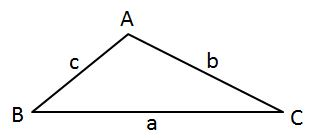#### Question 2 2. What's the value of sec2 (θ) given that the equation below is true?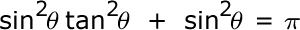#### Question 5 5. What is the value of cos(θ) given that the equation below is true?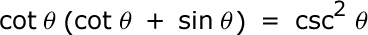### Page 2

#### Question 6 6. What is the area of the annulus/doughnut shown below?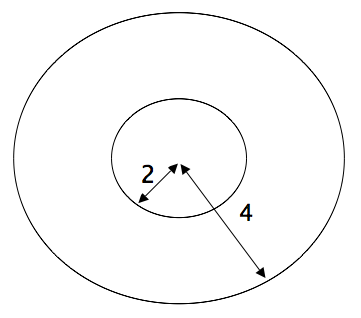#### Question 9 9. Simplify the following expression.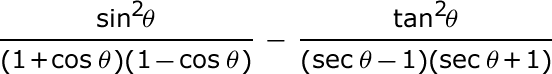#### Question 10 10. What is x?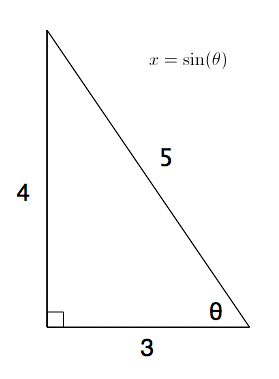### Page 3

#### Question 11 11. Which of the following is true?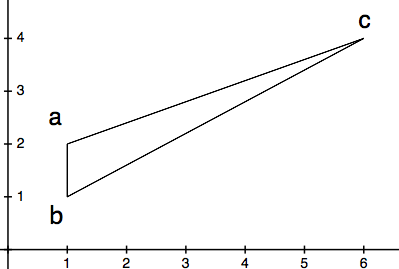#### Question 12 12. Simplify the following expression.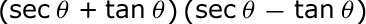#### Question 14 14. What is x?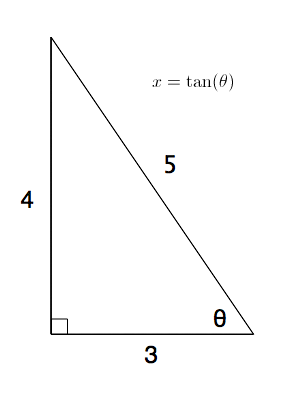#### Question 15 15. In the pictured triangle, side a is 23, side b is 18, and side c is 16. What is the approximate measure of ∠C? Round ∠C to the nearest whole number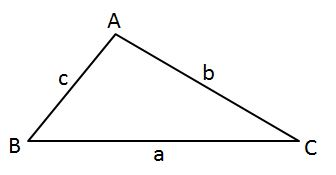### Page 4

#### Question 16 16. Jerry wants to buy a TV online, but the ad for the TV he wants to buy does not say what the screen size is. He knows that TV screens are measured diagonally. This image shows the length and width of the screen, so he decides to use the Pythagorean Theorem to determine the diagonal screen size. What is the screen size of the TV that Jerry wants to buy (to the nearest inch)?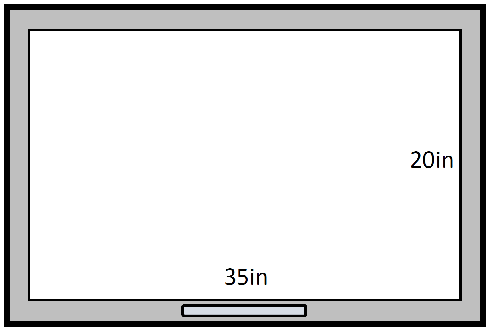#### Question 17 17. Find the value of x, rounded to the nearest degree.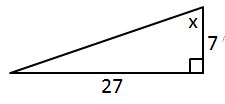### Page 5

#### Question 22 22. In the pictured triangle, what ratio represents the cosine of theta?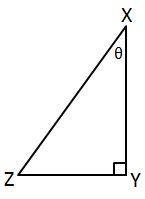#### Question 23 23. Which of these angles can replace x in tan (x)?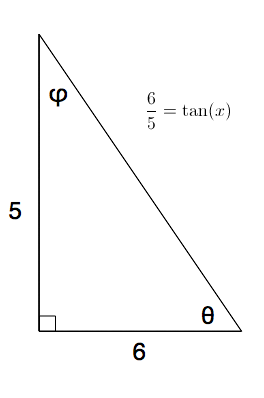#### Question 24 24. Find the value of x, rounded to the nearest tenth.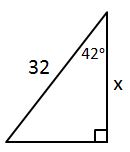#### Question 25 25. In the pictured triangle, side b is 12 and side c is 16. If ∠A is 84 degrees, then approximately how long is side a? Round the value of the cosine to the nearest whole number.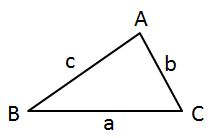### Page 6

#### Question 27 27. In the pictured triangle, ∠A is 58 degrees and ∠C is 63 degrees. If side a is 84 units long, approximately how long is side c?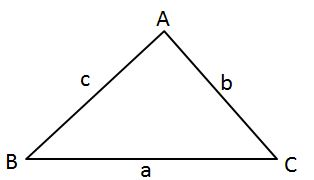#### Question 29 29. What is z in terms of x?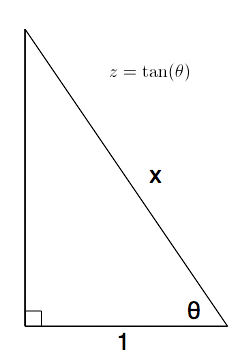#### Question 30 30. The lines a and b are parallel lines of length 5 and 2. The lines d and e are parallel to one another, and both are perpendicular to a and b. What is the length of c?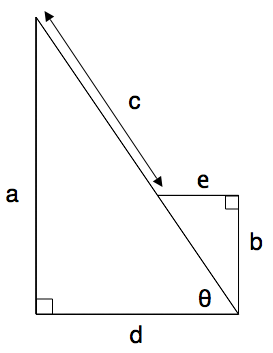#### High School Geometry: Introduction to Trigonometry Chapter Exam Instructions

Choose your answers to the questions and click 'Next' to see the next set of questions. You can skip questions if you would like and come back to them later with the yellow "Go To First Skipped Question" button. When you have completed the practice exam, a green submit button will appear. Click it to see your results. Good luck!

Support